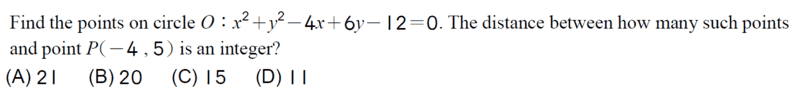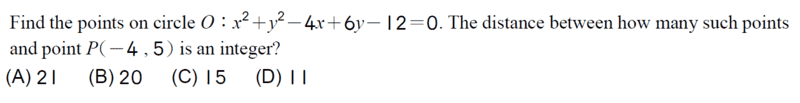# Find how many points on a circle have an integer distance from other points

songoku
Homework Statement:
Relevant Equations:
##(x-a)^2+(y-b)^2=r^2##

Distance between 2 pointsDistance between point (-4, 5) and point on circle:
$$d=\sqrt{(x+4)^2+(y-5)^2}$$
$$=\sqrt{x^2+8x+16+y^2-10y+25}$$

Then substitute ##y^2## from equation of circle:
$$d=\sqrt{x^2+8x+16-x^2+4x-6y+12-10y+25}$$
$$=\sqrt{12x-16y+53}$$

After this, I need to try the points one by one to check whether the distance is an integer and also whether the point is located on the circle? I am pretty sure there should be a more sane method to do this

Thanks

•Delta2

Homework Helper
2022 Award
I would start by changing coordinates to put the origin at circle center. Then there are only a few right triangles with integer sides. Actually I'll bet you can show it must be an even number,which is all you need for this.
More later if necessary .

•WWGD and songoku
songoku
Thank you very much hutchphd

Hall

Can you workout the center of the circle? We would get it as (2,-3). And radius?

Take any point on the circle, draw a triangle joining the center, the point (-4,5) and the arbitrary point on the circle (x,y). We know the distance between the point on circle and the center is ##r## (please workout it out) and the distance between the center and (-4,5) is 10 units. With resepct to the angle made at the center in this triangle, can you write out the cosine rule?

Homework Helper
2022 Award
With resepct to the angle made at the center in this triangle, can you write out the cosine rule
Or just "complete the square" to find the original radius.
I seem to be having trouble getting any of the prescribed answers although my symmetry argument seems correct. But I have been wrong before!

Homework Helper
Gold Member
2022 Award
First, a change of coordinates to ##\bar x = x - 2, \ \bar y = y + 3## is a good idea.

Then use the symmetry to move the point ##P## to somewhere simpler, keeping the same relationship with the circle.

Then count.

However, as the circle itself has an integer radius ...

•songoku
Homework Helper
2022 Award
It does? Jeez I must have screwed up. I wish I could do arithmetic.

Last edited:
Staff Emeritus
Homework Helper
Gold MemberAfter this, I need to try the points one by one to check whether the distance is an integer and also whether the point is located on the circle? I am pretty sure there should be a more sane method to do this

Thanks
No, you only need to check the distance for at most 2 points on the circle. Those being the point nearest to ##(-4,~5)## and the one farthest from ##(-4,~5)##.

How many integers fall between those two extremes?

•songoku, OmCheeto and hutchphd
Homework Helper
2022 Award
No, you only need to check the distance for at most 2 points on the circle. Those being the point nearest to (−4, 5) and the one farthest from (rs by symmetry−4, 5).
Except I believe the points (almost) always come in equidistant pairs...

Staff Emeritus
Homework Helper
Gold Member
Except I believe the points (almost) always come in equidistant pairs...
I don't claim that's the entire solution.

Let's leave something for song-o to figure out.

(Added later, just so you don't think I'm time traveling)

Yes, I also get one of the listed answers.

Last edited:
•songoku and hutchphd
Homework Helper
2022 Award
Did you get one of the multiple listed answers (we can do that much)?

Homework Helper
Gold Member
2022 Award
Did you get one of the multiple listed answers (we can do that much)?
Yes.

Homework Helper
2022 Award
I will look forward to the complete answer at the appropriate time ...!

EDIT DUH!: When you complete the square the quadratic terms are always positive. I wish I could do arithmetic. Thanks.

songoku
I got the answer using @hutchphd hint in post#2 but the method I use is not as efficient as @SammyS in post#8

But sorry I can't continue the hint given by @PeroK in post#6

Homework Helper
Gold Member
2022 Award
But sorry I can't continue the hint given by @PeroK in post#6
By symmetry, the answer must be an even number.

songoku
By symmetry, the answer must be an even number.
If all the options were even numbers, how to move point P to obtain the answer?

Thanks

Homework Helper
Gold Member
2022 Award
If all the options were even numbers, how to move point P to obtain the answer?
Rotate the system to put ##P## on the ##x##-axis.

•songoku
songoku
Thank you very much Hall, hutchphd, SammyS, PeroK

•Hall and hutchphd
Prof B
To calculate the answer you need the radius of the circle and the distance between P and the center of the circle. After that the coordinates are irrelevant.

•songoku and PeroK
•